Truncated cuboctahedron

﻿
Truncated cuboctahedron
Truncated cuboctahedron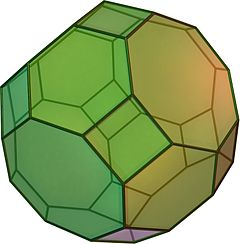Type Archimedean solid
Uniform polyhedron
Elements F = 26, E = 72, V = 48 (χ = 2)
Faces by sides 12{4}+8{6}+6{8}
Schläfli symbol t0,1,2{4,3}
Wythoff symbol 2 3 4 |
Coxeter-DynkinSymmetry Oh, [4,3], (*432)
Dihedral Angle
References U11, C23, W15
Properties Semiregular convex zonohedron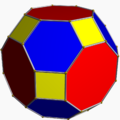Colored faces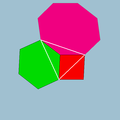4.6.8
(Vertex figure)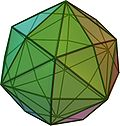Disdyakis dodecahedron
(dual polyhedron)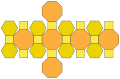Net

In geometry, the truncated cuboctahedron is an Archimedean solid. It has 12 square faces, 8 regular hexagonal faces, 6 regular octagonal faces, 48 vertices and 72 edges. Since each of its faces has point symmetry (equivalently, 180° rotational symmetry), the truncated cuboctahedron is a zonohedron.

Cartesian coordinates

The Cartesian coordinates for the vertices of a truncated cuboctahedron having edge length 2 and centered at the origin are all permutations of:

(±1, ±(1+√2), ±(1+2√2))

Area and volume

The area A and the volume V of the truncated cuboctahedron of edge length a are:$A = 12(2+\sqrt{2}+\sqrt{3}) a^2 \approx 61.7551724a^2$$V = (22+14\sqrt{2}) a^3 \approx 41.7989899a^3.$

Vertices

To derive the number of vertices, we note that each vertex is the meeting point of a square, hexagon, and octagon.

• Each of the 12 squares with their 4 vertices contribute 48 vertices because$12 \times 4=48$.
• Each of the 8 hexagons with their 6 vertices contribute 48 vertices because$8 \times 6=48$.
• Each of the 6 octagons with their 8 vertices contribute 48 vertices because$6 \times 8=48$.

Therefore, there may seem to exist 48 + 48 + 48 = 144 vertices. However, we have over-counted the vertices thrice since a square, hexagon, and octagon meet at each vertex. Consequently, we divide 144 by 3 to correct for our over-counting: 144 / 3 = 48.

Dual

If the original truncated cuboctahedron has edge length 1, its dual disdyakis dodecahedron has edge lengths$\frac{2}{7}\sqrt{30-3\sqrt{2}}$,$\frac{3}{7}\sqrt{6(2+\sqrt{2})}$ and$\frac{2}{7}\sqrt{6(10+\sqrt{2})}$.

Uniform colorings

There is only one uniform coloring of the faces of this polyhedron, one color for each face type.

A 2-uniform coloring also exists with alternately colored hexagons.

Other names

Alternate interchangeable names are:

The name truncated cuboctahedron, given originally by Johannes Kepler, is a little misleading. If you truncate a cuboctahedron by cutting the corners off, you do not get this uniform figure: some of the faces will be rectangles. However, the resulting figure is topologically equivalent to a truncated cuboctahedron and can always be deformed until the faces are regular.

The alternative name great rhombicuboctahedron refers to the fact that the 12 square faces lie in the same planes as the 12 faces of the rhombic dodecahedron which is dual to the cuboctahedron. Compare to small rhombicuboctahedron.

One unfortunate point of confusion: There is a nonconvex uniform polyhedron by the same name. See nonconvex great rhombicuboctahedron.

Wikimedia Foundation. 2010.

Look at other dictionaries:

• Great truncated cuboctahedron — In geometry, the great truncated cuboctahedron is a nonconvex uniform polyhedron, indexed as U20. Cartesian coordinates Cartesian coordinates for the vertices of a great truncated cuboctahedron centered at the origin are all permutations of: (±1 …   Wikipedia

• Cuboctahedron — (Click here for rotating model) Type Archimedean solid Uniform polyhedron Elements F = 14, E = 24, V = 12 (χ = 2) Faces by sides 8{3}+6{4} …   Wikipedia

• Truncated octahedron — (Click here for rotating model) Type Archimedean solid Uniform polyhedron Elements F = 14, E = 36, V = 24 (χ = 2) Faces by sides 6 …   Wikipedia

• Truncated icosidodecahedron — (Click here for rotating model) Type Archimedean solid Uniform polyhedron Elements F = 62, E = 180, V = 120 (χ = 2) Faces by sides …   Wikipedia

• Cubitruncated cuboctahedron — Type Uniform star polyhedron Elements F = 20, E = 72 V = 48 (χ = −4) Faces by sides 8{6}+6{8}+6{8/3} Wythoff …   Wikipedia

• Runcinated tesseract — Tesseract …   Wikipedia

• Uniform star polyhedron — A display of uniform polyhedra at the Science Museum in London …   Wikipedia

• Snub cube — (Click here for rotating model) Type Archimedean solid Uniform polyhedron Elements F = 38, E = 60, V = 24 (χ = 2) Faces by sides (8+24){3}+6{4} …   Wikipedia

• Liste Des Polyèdres Uniformes — Les polyèdres uniformes et les pavages forment un groupe bien étudié. Ils sont listés ici pour une comparaison rapide de leurs propriétés et de leurs noms de schéma variés ainsi que de leurs symboles. Cette liste inclut : tous les 75… …   Wikipédia en Français

• Liste des polyedres uniformes — Liste des polyèdres uniformes Les polyèdres uniformes et les pavages forment un groupe bien étudié. Ils sont listés ici pour une comparaison rapide de leurs propriétés et de leurs noms de schéma variés ainsi que de leurs symboles. Cette liste… …   Wikipédia en Français